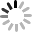铝用炭素配方技术

1 多元线性回归配方计算方法的原理解析

f 工 (x)=b 1 f 大 (x)+b 2 f 小 (x) = f 理 (x) （ 1 ）E 2 =∑(b 1 f 大 (x)+b 2 f 小 (x)-f 理 (x)) 2 （ 2 ）需要注意的是，配方问题中各级原料配入比例之和为 100% ， 这是一个典型的带线性约束的二元线性回归方程  的求解问题。 限制条件如式（ 3 ）所示：b 1 +b 2 =1 （ 3 ）这类问题中， 若要保证限制条件的第一有效性，即工作配方结果中式（ 3 ）绝对成立，可通过在限制条件两端乘以一个较大的系数来实现，系数大小可根据数据源的数量级来确定 [6-7] 。 配方问题中数据都是以“ % ”为单位的十位级，可在式（ 3 ）两端乘以系数 1000 ，得到式（ 4 ）：1000b 1 +1000b 2 =1000 （ 4 ）

2 实际配方问题的处理

2.1 用传统方法求解实际配方

① 粗料仓用量： 11/73×100%=15.1%

② 中料仓用量：（ 14-15.1×12.7% ） /89.4×100%=

11.1%

③ 粉料仓用量：（ 58±3 ） % ，取 55% 。

④ 余量： 1-15.1%-11.1%-55%=18.8%

⑤ 将余量按一定比例分配到粗、中、细、粉四级

2.2 用多元线性回归法求解实际配方

f 理 (x i )= b 1 f 粗 (x i )+b 2 f 中 (x i )+b 3 f 细 (x i )+b 4 f 粉 (x i )(i=1,2,3,4,5) （ 5 ）式（ 5 ）中： x i —— — 颗粒粒径， mm ； b i —— — 各级原料的配料比例。

1000b 1 +1000b 2 +1000b 3 +1000b 4 =1000 （ 6 ）理论配方要求粒径大于 4 mm 的颗粒比例小于2% ，不妨令其为 1% 。 将表 1 和表 2 结合，加入限制条件（式 6 ），整理得到软件计算用数据总表（表 4 ）。

（ 1 ） MATLAB

n=6;m=4;

y=[1 11 14 58 43 1000];

x1=[8.7 73 12.7 0 0 1000];

x2=[0 1 89.4 0 0 1000];

x3=[0 0 2 36.5 6.5 1000];

x4=[0 0 0 100 77 1000];

X=[ x1 ’ ,x2 ’ ,x3 ’ ,x4 ’ ];

b=regress(y`,X)

b= 0.155

0.136

0.182

0.527

（ 2 ） SPSS

（ 3 ） EXCEL

3 结论

6 。

3.2 燃气消耗对比分析

4 结束语